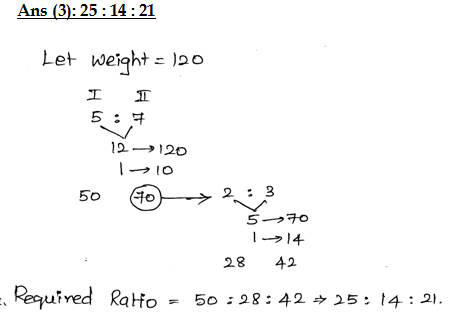# Ratio and Proportion Questions Set-1 for Bank Exam

R atios and Proportions quiz for bank pre exams : Ratios and Proportions is a major part of quantitative aptitude in banking exams of IBPS, SBI, RBI PO and Clerk post. Ratios and Proportions is just about practice and shortcut that one gets to know during the practice itself. Ratios and Proportions is something that you need to practice a lot. So, we are providing Ratios and Proportions quiz based on examination pattern of IBPS, SBI, RBI PO and Clerk exam, for the aspirants to prepare it efficiently. ThisRatios and Proportions quiz is designed in a way to be very interesting to the candidates. Here, Ratios and Proportions answers and detailed solutions are also provided. Solving these questions will help you save time in IBPS, SBI and RBI PO and Clerk exam and give time to other questions.
Ratios and Proportions Quiz to improve your Quantitative Aptitude for SBI Po & SBI clerk exam IBPS PO Reasoning , IBPS Clerk Reasoning , IBPS RRB Reasoning, LIC AAO ,LIC Assistant and other competitive exam

## Ratio and Proportion

1➤ If the ratio of incomes of A and B in 2001 is 2 : 3 and the ratio of incomes of A in 2001 and 2002 is 4 : 5. Find the expenditure of A in 2002, if saving in the same year is Rs.4000. It is given that in 2001 the sum of income of A and B is Rs.25000.
(1) Rs.5000
(2) Rs.10500
(3) Rs.9500
(4) Rs.7500
(5) Rs.8500

=>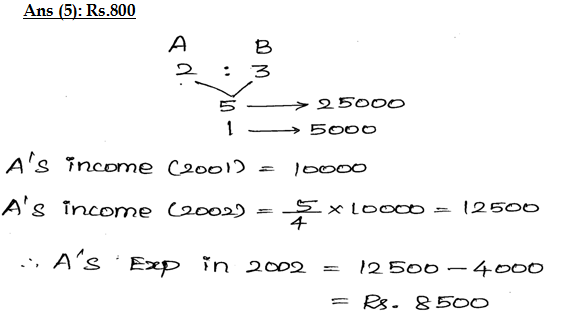2➤ Rs.33630 are divided among A, B and C in such a manner that the ratio of the amount of A to that of B is 3 : 7 and the ratio of the amount of B to that of C is 6 : 5. The amount of money received by B is
(1) Rs.14868
(2) Rs.16257
(3) Rs.13290
(4) Rs.12390
(5) None of these

=>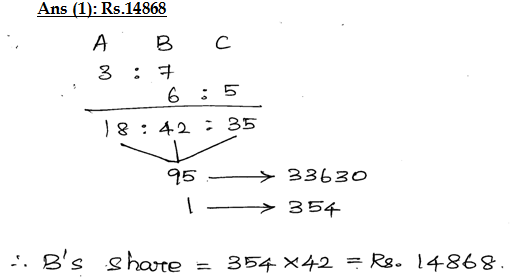3➤ A man leaves Rs.8600 to be divided among 5 sons, 4 daughters and 2 nephews, if each daughter receives four times as much as each nephew and each son receives five times as much as each nephew, how much does each daughter receive?
(1) 100
(2) 600
(3) 800
(4) 1000
(5) None of these

=>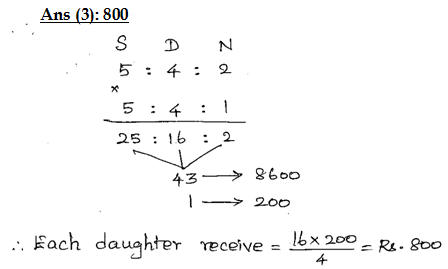4➤ Amounts invested by Ajith and Suresh are in the ratio of 4 : 7 respectively. If the total amount invested by the persons together is Rs.49,500, what is the amount invested by Suresh?
(1) Rs.31,500
(2) Rs.18,000
(3) Rs.31,000
(4) Rs.18,500
(5) None of these

=>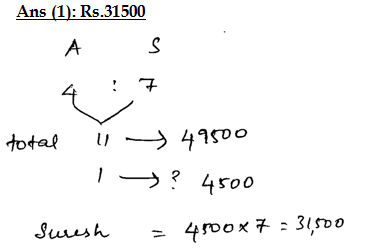5➤ A watermelon is cut into 2 pieces as their weight are in ratio of 5 : 7. The bigger part is also cut into two places as their weight are in ratio 2 : 3. Calculate the ratio of the weight of final three places.
(1) 25 : 9 : 18
(2) 21 : 25 : 10
(3) 25 : 14 : 21
(4) 13 : 23 : 27
(5) None of these

=>